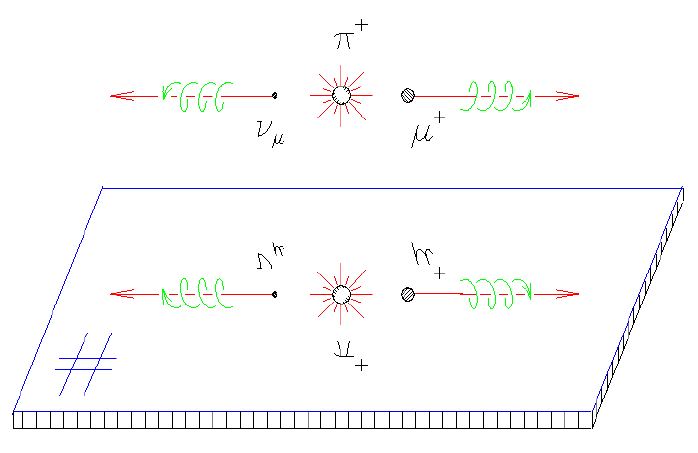# Pion Decay and its Mirror Image

The positive pion, a spinless particle, initially has zero angular momentum and zero linear momentum (we consider only pion decay at rest). Therefore linear momentum conservation requires that the decay products (a positive muon and a muon neutrino) are emitted in opposite directions with equal and opposite momenta; meanwhile, angular momentum conservation requires that they have equal and opposite spin. The weak interaction governing this decay process has the remarkable property that it creates only ``left-handed'' or ``negative helicity'' neutrinos (i.e. having their spin and angular momentum in opposite directions, as shown) and ``right-handed'' or ``positive helicity'' antineutrinos, so the µ+ must also have its spin pointing back along its momentum. This gives a beam of perfectly spin-polarized muons from pion decay - an essential ingredient for µSR.The mirror-image reaction never occurs in nature, because the parity inversion performed by the mirror changes a left-handed neutrino to a right-handed one, which the weak interaction cannot produce. If, however, the P (parity) operator is combined with a C (charge conjugation - changing all particles to their corresponding antiparticles) operator, the positive charges change to negative ones and the neutrino changes to an antineutrino, which is allowed (in fact required) to have positive helicity (be right-handed) and the reaction thus altered is allowed as much as the original one. These features are now well known, but in 1957 when they were confirmed it was a veritable cataclysm in theoretical physics, which had always considered ``parity symmetry'' to be a universal law of nature!
Jess H. Brewer